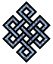#fail2ban bad ip database: ip 182.231.203.182

### | ip database | live view | stats | report | help | api key:

 ip: 182.231.203.182 hostname: 182.231.203.182 country:[KR] Korea, Republic of first reported: 27.07.2017 11:23.51 GMT+0200 last reported: 07.03.2019 09:51.31 GMT+0200 time period: 587d 23h 27m 40s total reports: 7 reported by: 2 host(s) filter(s): ssh (4) ssh (3) tor exit node no badips.com db Lookup## port scan of '182.231.203.182':

[-hide]
```# Nmap 6.40 scan initiated Thu Jul 27 11:24:02 2017 as: /usr/bin/nmap -sU -sS -O 182.231.203.182
Nmap scan report for 182.231.203.182
Host is up (0.31s latency).
Not shown: 1000 open|filtered ports, 992 filtered ports
PORT     STATE  SERVICE
2121/tcp open   ccproxy-ftp
2222/tcp open   EtherNet/IP-1
3689/tcp open   rendezvous
8080/tcp open   http-proxy
8090/tcp open   unknown
8200/tcp closed trivnet1
9080/tcp open   glrpc
9091/tcp open   xmltec-xmlmail
No exact OS matches for host (If you know what OS is running on it, see http://nmap.org/submit/ ).
TCP/IP fingerprint:
OS:SCAN(V=6.40%E=4%D=7/27%OT=2121%CT=8200%CU=%PV=N%G=Y%TM=5979B177%P=x86_64
OS:-pc-linux-gnu)SEQ(SP=102%GCD=2%ISR=10C%TI=I%CI=Z%TS=U)OPS(O1=M5B4NNSNW9%
OS:O2=M5B4NNSNW9%O3=M5B4NW9%O4=M5B4NNSNW9%O5=M5B4NNSNW9%O6=M5B4NNS)WIN(W1=3
OS:908%W2=3908%W3=3908%W4=3908%W5=3908%W6=3908)ECN(R=Y%DF=N%TG=40%W=3908%O=
OS:M5B4NNSNW9%CC=N%Q=)T1(R=Y%DF=N%TG=40%S=O%A=S+%F=AS%RD=0%Q=)T2(R=N)T3(R=N
OS:)T4(R=Y%DF=Y%TG=40%W=0%S=A%A=Z%F=R%O=%RD=0%Q=)T5(R=Y%DF=Y%TG=40%W=0%S=Z%
OS:A=S+%F=AR%O=%RD=0%Q=)T6(R=Y%DF=Y%TG=40%W=0%S=A%A=Z%F=R%O=%RD=0%Q=)T7(R=N
OS:)U1(R=N)IE(R=N)

OS detection performed. Please report any incorrect results at http://nmap.org/submit/ .
# Nmap done at Thu Jul 27 11:25:11 2017 -- 1 IP address (1 host up) scanned in 70.40 seconds
```
```Σ = 108 | Δt = 0.0061089992523193s
```